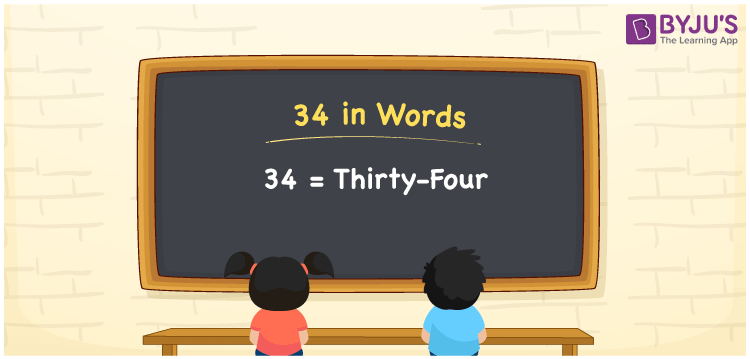# 34 in Words

Thirty-four is the number name of the number 34. Using the place value of a given number, it is simple to convert numbers to words. As a result, while writing numerical names, the place value chart is quite important. If you spent Rs. 34 on a piece of chocolate, for example, you may write “I spent Rs. Thirty-four on a piece of chocolate”. We’ll learn how to write the number 34 in words as well as how to spell it in English in this article.

 34 in Words: Thirty-four Thirty-four in Numerical Form: 34

## 34 in English Words## How to Write 34 in Words?

The place value chart for the number 34 is shown below. To write the number name, first, get the number’s place value and then convert the number to words.

 Tens Ones 3 4

The expanded form of the number 34 is shown below.

= 3 × Ten + 4 × One

= 3 × 10 + 4 × 1.

= 30 + 4

= 34

= thirty-four

Hence, 34 in words is thirty-four.

The natural number 34 occurs before 35 but after 33.

34 in words – Thirty-four

Is 34 an odd number? – No

Is 34 an even number? – Yes

Is 34 a perfect square number? – No

Is 34 a perfect cube number? – No

Is 34 a prime number? – No

Is 34 a composite number? – Yes

## Frequently Asked Questions on 34 in Words

Q1

### How to write 34 in words?

34 in words is thirty-four.

Q2

### Simplify 40 – 6, and express in words.

Simplifying 40 – 6, we get 34. Hence, 34 in words is thirty-four.

Q3

### Convert thirty-four into numbers.

Thirty-four in numbers is 34.